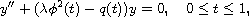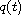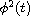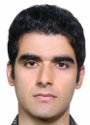Electron. J. Diff. Equ., Vol. 2013 (2013), No. 182, pp. 1-16.

### Reconstructing the potential function for indefinite Sturm-Liouville problems using infinite product forms Mohammad Dehghan, Ali Asghar Jodayree

Abstract:
In this article we consider the linear second-order equation of Sturm-Liouville typewhereis a real parameter,is the potential function andis the weight function. We use the infinite product representation of the derivative of the solution to the differential equation with Dirichlet-Neumann conditions, and for the system of dual equations which is needed for expressing inverse problem and for retrieving potential. It must be mentioned that the weight function has a zero whose order is an integer called a turning point.

Submitted July 15, 2013. Published August 7, 2013.
Math Subject Classifications: 34B24, 34A55, 34E20, 34E05.
Key Words: Indefinite Sturm-Liouville problem; turning point; dual equations; infinite product form.

Show me the PDF file (288 KB), TEX file, and other files for this article.Mohammad Dehghan Faculty of Mathematical Sciences University of Tabriz Tabriz, Iran email: m-dehghan@tabrizu.ac.ir, Tel. +989113512619 Ali Asghar Jodayree Faculty of Mathematical Sciences University of Tabriz Tabriz, Iran email: akbarfam@yahoo.com